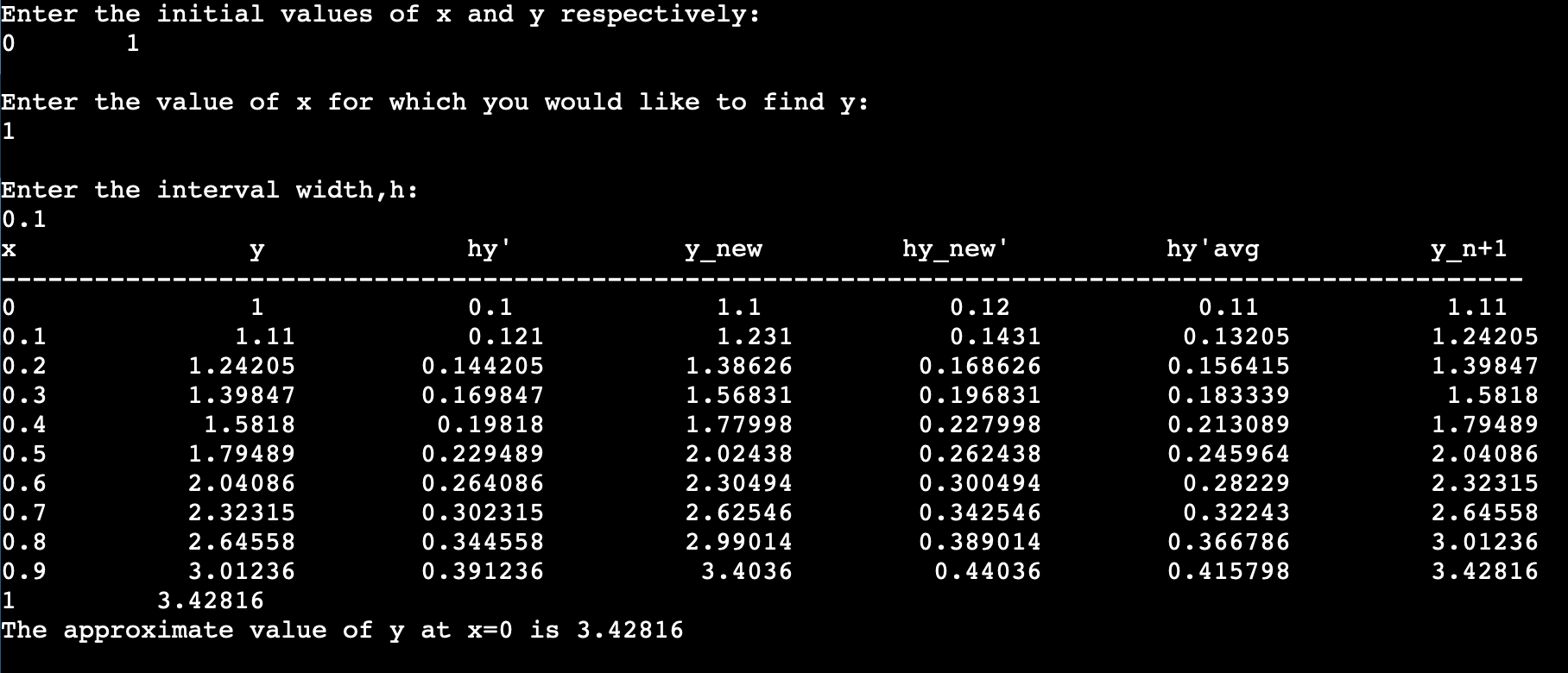# C++ Program for Modified Euler’s Method to solve an ODE

### Run the code online here:

https://onlinegdb.com/N-6KusNbR

### Code:

```//Modified Euler's Method for differential equations
#include<iostream>
#include<math.h>
#include<iomanip>
using namespace std;
double df(double x, double y)
{
double a=x+y;            //function for defining dy/dx
return a;
}
int main()
{
double x0,y0,x,y_i,dy1,dy2,dy_avg,y_n,h;    //for initial values, width, etc.
cout<<"\nEnter the initial values of x and y respectively:\n"; cin>>x0>>y0;                    //Initial values
cout<<"\nEnter the value of x for which you would like to find y:\n"; cin>>x;
cout<<"\nEnter the interval width,h:\n"; cin>>h;                        //input width
cout<<"x"<<setw(16)<<"y"<<setw(16)<<"hy'"<<setw(16)<<"y_new"<<setw(16)<<"hy_new'"<<setw(16)<<"hy'avg"<<setw(16)<<"y_n+1"<<endl;
cout<<"--------------------------------------------------------------------------------------------------\n";
while(fabs(x-x0)>0.0000001)      //I couldn't just write "while(x0<x)" as they both are floating point nos. It is dangerous to compare two floating point nos. as they are not the same in binary as they are in decimal. For instance, a computer cannot exactly represent 0.1 or 0.7 in binary just like decimal can't represent 1/3 exactly without recurring digits.
{
dy1=h*df(x0,y0);        //calculate slope or dy/dx at x0,y0
y_i=y0+dy1;            //calculate new y, which is y0+h*dy/dx
dy2=h*df(x0+h,y_i);        //calculate slope or dy/dx at x0+h (new x),new y
dy_avg=(dy1+dy2)/2.0;        //calculate the average of the slopes at y0 and new y
y_n=y0+dy_avg;            //calculate new y, which is y0+h*average(dy/dx)
cout<<x0<<setw(16)<<y0<<setw(16)<<dy1<<setw(16)<<y_i<<setw(16)<<dy2<<setw(16)<<dy_avg<<setw(16)<<y_n<<endl;
x0=x0+h;            //calculate new x.
y0=y_n;                //pass this new y as y0 in the next iteration.
}
cout<<x0<<setw(16)<<y0<<endl;
cout<<"The approximate value of y at x=0 is "<<y0<<endl;    //print the solution.
return 0;
}```

### Run the code online here:

https://onlinegdb.com/N-6KusNbR

### Sample output### Exact (ideal) solution

Explaination of the code:[wpedon id="7041" align="center"]